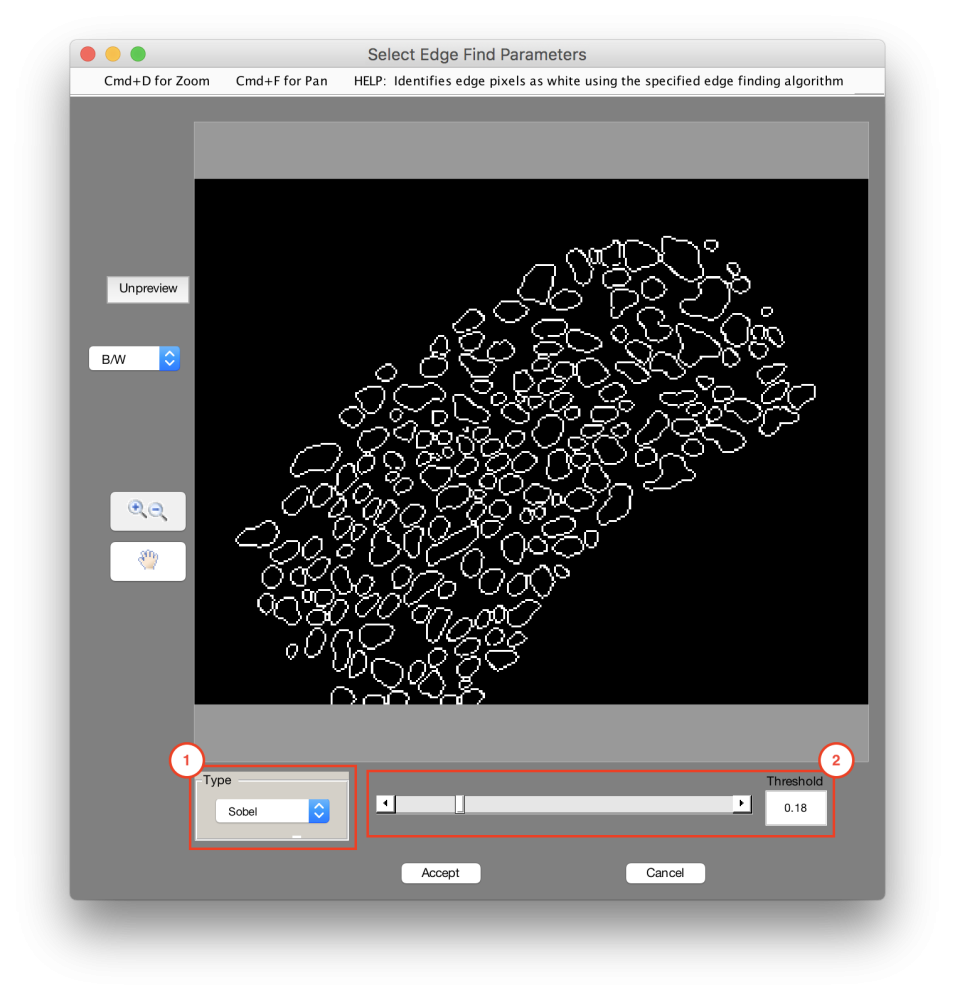### Segmentation > Find Edges

Sets edge pixels as empty using the specified edge finding algorithm [1-3].### 1. Type

The edge finding algorithm used

• Sobel: Detect edges using the Sobel algorithm
• Prewitt: Detect edges using the Prewitt algorithm
• Roberts: Detect edges using the Roberts algorithm
• Laplacian: Detect edges using the Laplacian algorithm
• Zero-Cross: Detect edges using the Zero-Cross algorithm
• Canny: Detect edges using the Canny algorithm (tends to cleaner edges than other algorithms) 

### 2. Threshold

A weakness setting for the edge find algorithm. Higher thresholds are weaker edge finds, but maybe produce less noise. (Recommended: 0.01)

#### Tips

• It is often useful to optimize the Find Edges function to a Standard Deviation Filter companion image. This allows for dynamic edge finding in presence of variation.

## Referrences

 Canny, John, “A Computational Approach to Edge Detection,” IEEE Transactions on Pattern Analysis and Machine Intelligence,Vol. PAMI-8, No. 6, 1986, pp. 679-698.

 Lim, Jae S., Two-Dimensional Signal and Image Processing, Englewood Cliffs, NJ, Prentice Hall, 1990, pp. 478-488.

 Parker, James R., Algorithms for Image Processing and Computer Vision, New York, John Wiley & Sons, Inc., 1997, pp. 23-29.

Need more help with this?
Chat with an expert now ››